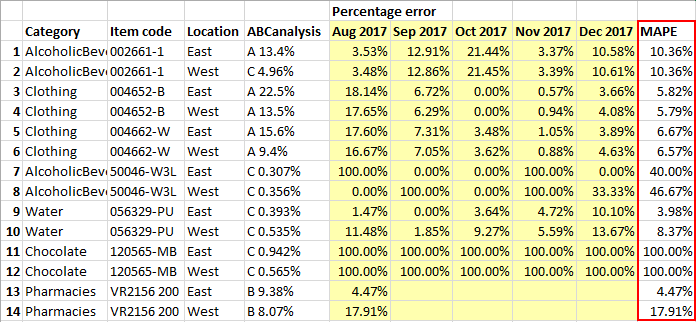rolling-forecast

# 4.11. Rolling Forecast

Sometimes there is a need to know how the forecast suggested by a system or proposed by a planner differs from the actual data as times goes by.

For example, you need to anticipate sales or revenues half a year in advance. After the first month had passed, you drop that month from the beginning of the forecast and add another month to the end of the forecast. Such a forecast is called rolling forecast. The technique relies on an add/drop approach to forecasting that creates new forecast periods on a rolling basis. A rolling forecast's first in/first out (FIFO) process ensures that the forecast always covers the same amount of time (see Figure 1 below).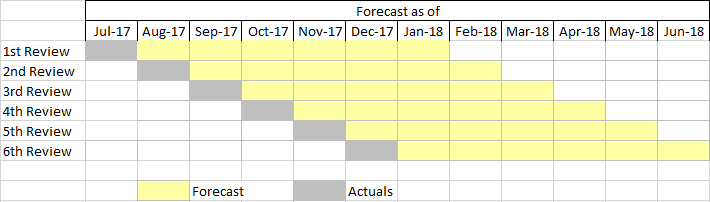Figure 1. Rolling forecast

Then, there is a time to evaluate a rolling forecast in order to analyze the accuracy of the forecasts. That can be done, for example, on a yearly bases.

## Evaluating Rolling Forecast

There are many measures used to evaluate the error (or accuracy) of rolling forecast. Among them:

• mean squared error (MSE);
• root mean squared error (RMSE);
• mean absolute error (MAE); or
• mean absolute percentage error (MAPE).

They can be used to evaluate rolling forecast for one, two, or more steps ahead. For example, if we need to evaluate rolling forecast one month ahead using MAPE within the period from Aug 2017 to Dec 2017 (see Figure 1), the formula looks like:,

where `At` and `Ft` - the actual and forecast data for the cells highlighted in Figure 2.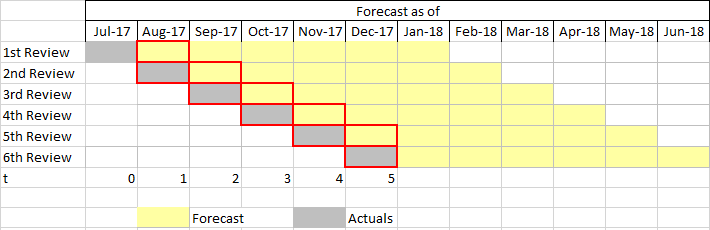Figure 2. Evaluating rolling forecast

## Rolling Forecast in Streamline

Streamline implements rolling forecast by predicting the future over a set period of time.

Consider an example. Assume that today is the end of Jul 2017 and we build forecasts 6 months ahead (see figure below).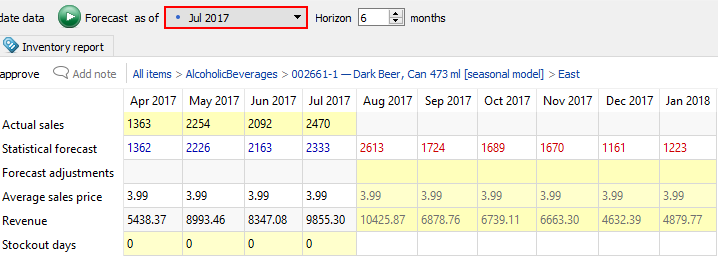After the forecasts have been generated, Streamline automatically assigns it to the current period. At the end of the next month – Aug 2017 – we generate the forecasts again. And so on. Let us do this until the end of Dec 2017. In the result, we have forecasts for 5 periods ranging from Aug 2017 to Dec 2017. You can see all the periods you generated forecasts for in the Period drop-down of the Final forecast report (see figure below).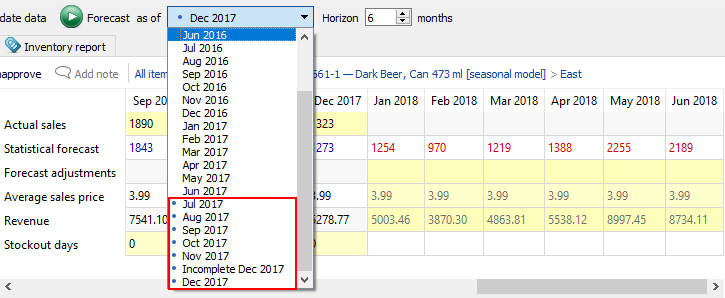To correlate rolling forecast in Streamline with the rolling forecast described in the first part of this article, a forecast generated for the As of period in Streamline corresponds to a Review in Figure 1. By selecting a particular period in the As of list, we can go back into the history of rolling forecast and see the forecasts generated at that time.

## Evaluating Rolling Forecast in Streamline

Let us proceed with the example described in the section above.

Streamline uses APE and MAPE to evaluate the forecast against actual data. Let us show you how to evaluate rolling forecast one month ahead within five months (from Aug 2017 to Dec 2017) in Streamline.

At the end of Dec 2017 we have all necessary actual data to evaluate the rolling forecast. Let us look at the item Dark Beer in the East location in the Jul 2017 period (see figure below).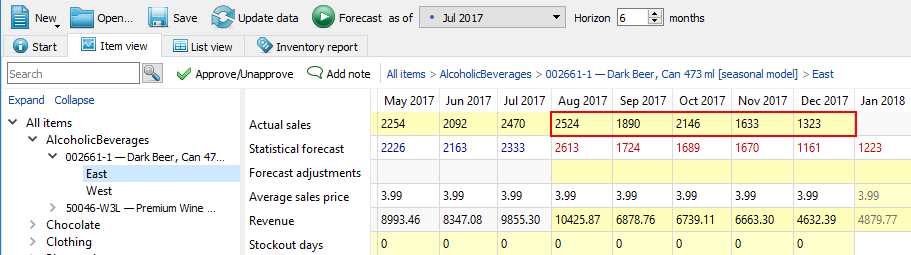As you see, Streamline automatically loads and shows all available actual data in the period selected in the As of control. It also evaluates the forecasts against the loaded actual data.

Forecast evaluation results are shown in the Forecast error report on the Reports tab. The Percentage error section of the report shows APE for each period/item/location. Thus, Aug 2017 column shows APE for one month ahead, Sep 2017 column indicates APE for two months ahead, and so on (see figure below).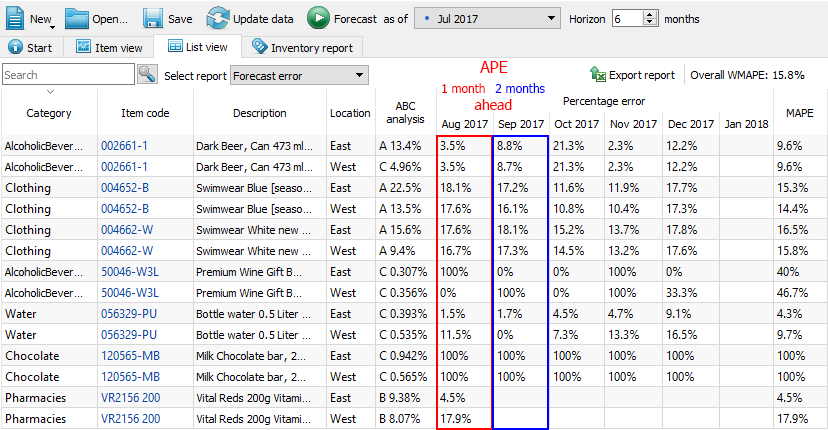To evaluate the rolling forecast one month ahead using MAPE, we need to take data from the first column of the Forecast error report across all the periods of the rolling forecast by selecting the particular period in the As of control.

Here are the steps to follow in order to evaluate the rolling forecast:

1. Export the Forecast error report for the first period (Jul 2017 in our case) of the rolling forecast.

1.1. Select the first period of the rolling forecast in the As of control.
1.2. Go to the Reports tab and select the Forecast error report.
1.3. Export the report by clicking the Export report button found on the toolbar of the Reports tab. The exported Excel table is shown in the figure below.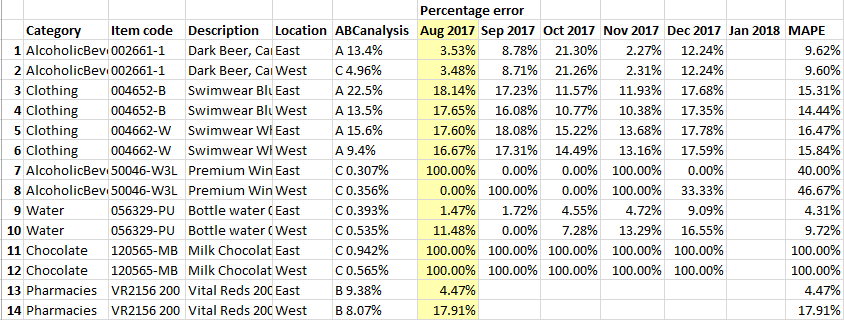The column highlighted in the figure above has the data needed to calculate MAPE for the rolling forecast.

2. Save the exported file under a different name, for instance, “Rolling forecast.xlsx”.
3. Export Forecast error report for the next period of the rolling forecast (Aug 2017 in our case).
4. Copy the data of the first period of the exported report (Sep 2017 column in our case) and paste it into the corresponding column of the first exported report table (see figure below).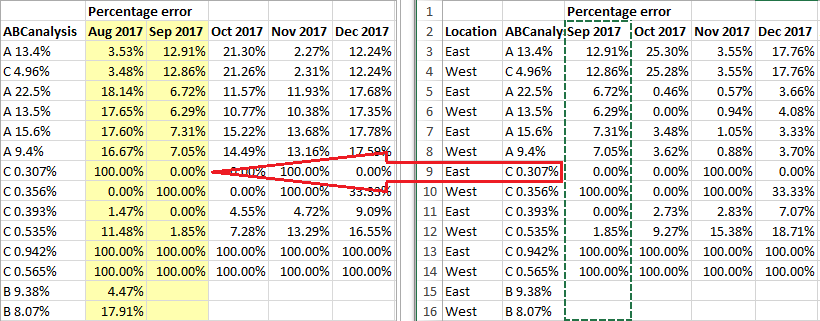5. Do 3-rd and 4-th steps for all the periods left. In our case, those are Sep 2017, Oct 2017, and Nov 2017 (see figure below).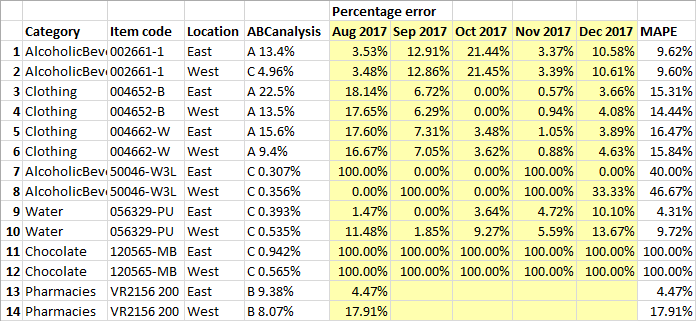6. Now, let us calculate MAPE for the rolling forecasts. To do this:

6.1. Edit the first cell of the MAPE column.
6.2. Type “=”, click the Insert function button, choose the AVERAGE function, and select the periods' data to average (see figure below).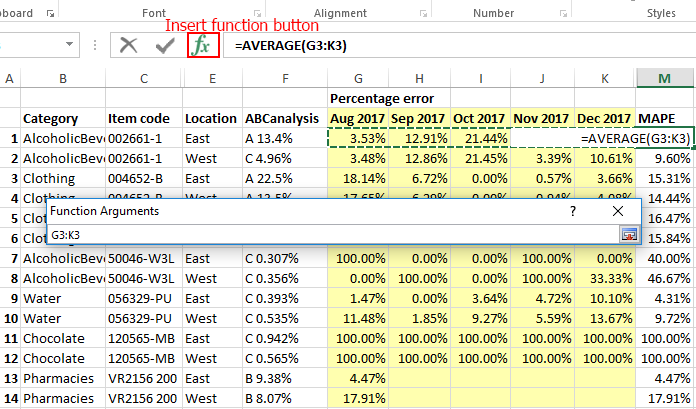6.3. Propagate the entered formula to the last item (see figure below).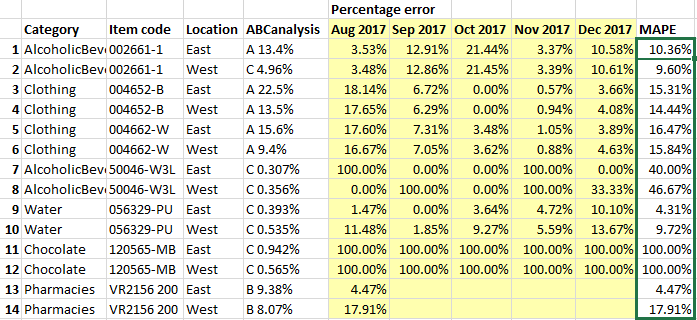6.4. The resultant evaluation of the rolling forecasts is shown in the figure below.